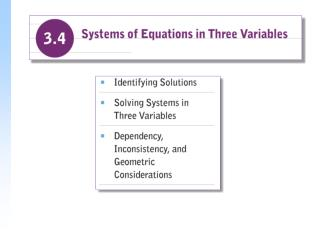# Identifying Solutions - PowerPoint PPT PresentationDownload PresentationIdentifying Solutions

Presentation Description
Download Presentation## Identifying Solutions

- - - - - - - - - - - - - - - - - - - - - - - - - - - E N D - - - - - - - - - - - - - - - - - - - - - - - - - - -
##### Presentation Transcript

1. Example Determine whether (2, –1, 3) is a solution of the system: Identifying Solutions A linear equation in three variables is an equation equivalent to one in the form Ax + By + Cz = D, where A, B, C,and D are real numbers. We refer to the form Ax + By + Cz = D as standard form for a linear equation in three variables. A solution of a system of three equations in three variables is an ordered triple (x, y, z) that makes all three equations true. Solution We substitute (2, –1, 3) into the three equations, using alphabetical order: – 4x + y + 2z = –3 x + y + z = 4 2x –2y – z = 3 – 4(2)+(–1) + 2(3) –3 2+ (–1) +34 2(2)– 2(–1) –33 TRUE 4 = 4 TRUE –3 = –3 3 = 3 TRUE The triple makes all three equations true, so it is a solution of the system.

2. Solving Systems in Three Variables The elimination method allows us to manipulate a system of three equations in three variables so that a simpler system of two equations in two variables is formed. Once that simpler system has been solved, we can substitute into one of the three original equations and solve for the third variable.

3. Example Solve the following system of equations: (1) (2) (3) Solution We select any two of the three equations and work to get one equation in two variables. Let’s add equations (1) and (2): (1) (2) (4) Adding to eliminate z 2x + 3y = 8 Next, we select a different pair of equations and eliminate the same variable. Let’s use (2) and (3) to again eliminate z. Multiplying equation (2) by 3 3x + 6y – 3z = 6 x – y + 3z = 8 4x + 5y = 14 (5)

4. Now we solve the resulting system of equations (4) and (5). That will give us two of the numbers in the solution of the original system, 2x + 3y = 8 (4) 4x + 5y = 14 (5) We multiply both sides of equation (4) by –2 and then add to equation (5): –4x – 6y = –16 4x + 5y = 14 –y = –2 y = 2 Substituting y = 2 into either equation (4) or (5) we get, 4x + 5(2) = 14 4x + 10 = 14 4x= 4 x = 1 Now we have x = 1 and y = 2. To find the value for z, we use any of the three original equations and substitute to find the third number z.

5. Let’s use equation (1) and substitute our two numbers in it: x + y + z = 6 1 + 2 + z = 6 z = 3 We have obtained the ordered triple (1, 2, 3). It should check in all three equations. The solution is (1, 2, 3).

6. Dependency, Inconsistency, and Geometric Considerations The graph of a linear equation in three variables is a plane. Solutions are points common to the planes of each system. Since three planes can have an infinite number of points in common or no points at all in common, we need to generalize the concept of consistency in three dimensions.

7. Example (1) (2) (3) Solve the following system of equations: Since we ended up with a false equation, or contradiction, we know that the system has no solution. The system is inconsistent. Solution The variable x is missing in equation (1). Let’s add equations (2) and (3) to get another equation with x missing: (2) (3) (4) Adding y + 2z = 6 Equations (1) and (4) form a system in y and z. Solve as before: Multiplying equation (1) by –1 y + 2z = 2 –y – 2z = –2 y + 2z = 6 y + 2z = 6 0 = 4

8. Examples: Solve each system